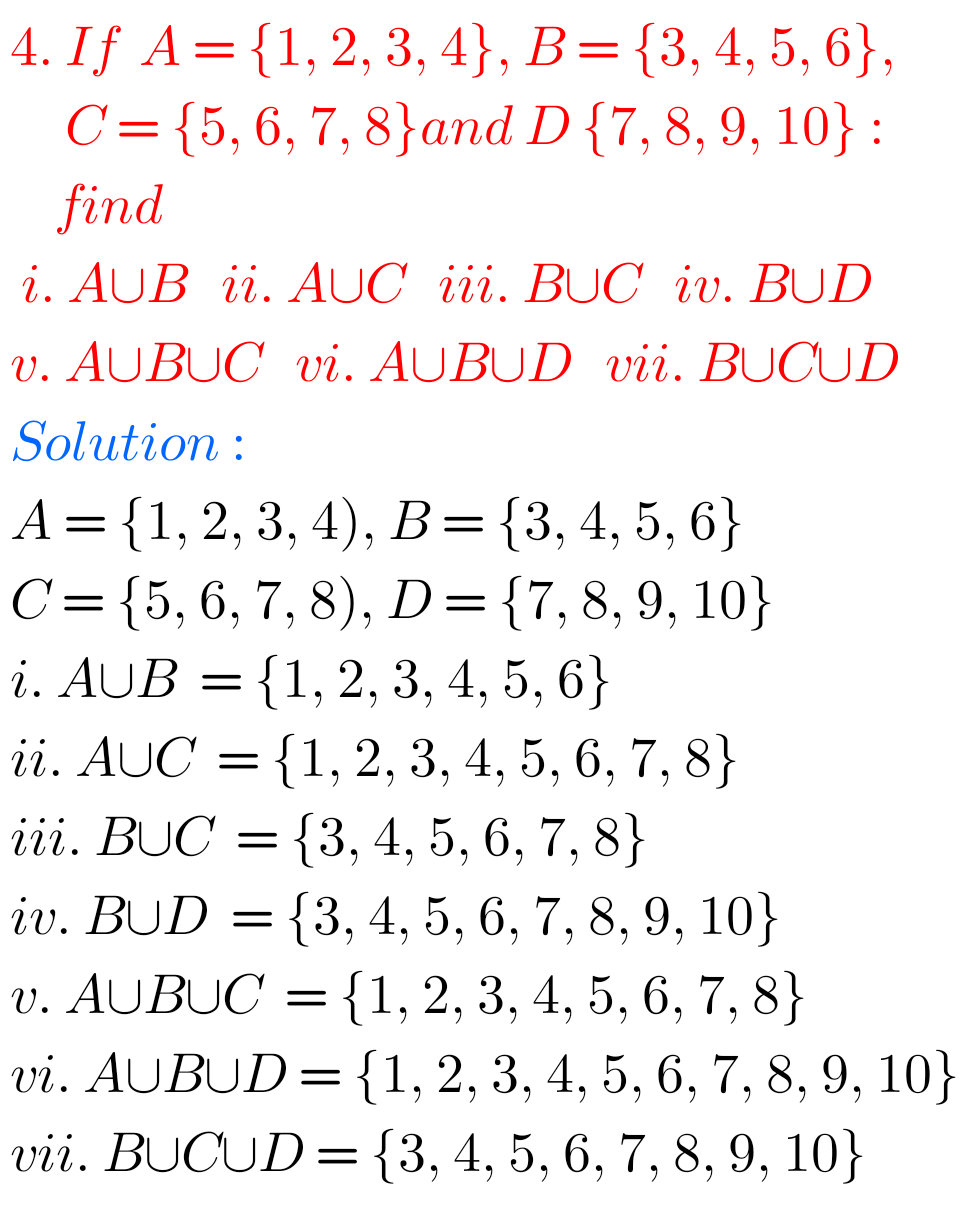## NCERT Class 11 maths Chapter 1 Sets exercise 1.4 solutions

Sets Exercise 1.4 solutions Class 11 NCERT maths NCERT Mathematics class 11 Chapter 1 Sets Exercise 1.4 Solutions are given. You should study the textbook lesson Sets very well. You should practice example problems and solutions are given in the textbook. Observe the solutions given below and try them in your own method. Sets Exercise …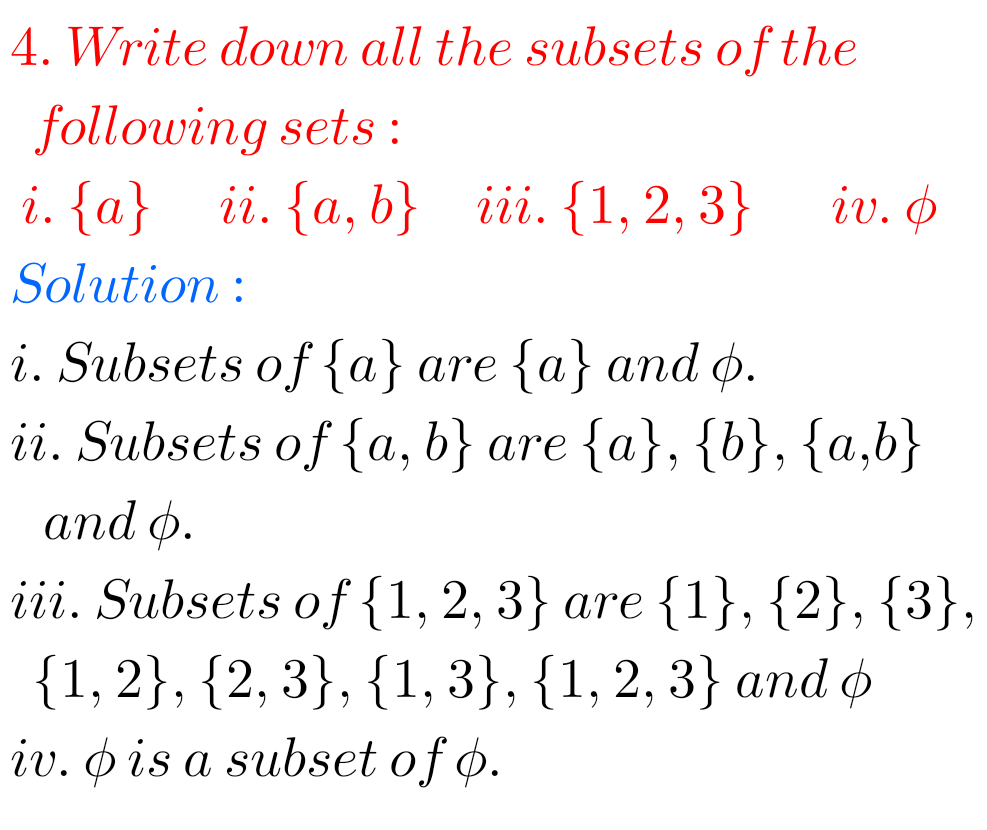## NCERT Maths solutions class 11 Chapter 1 Sets Exercise 1.3

Sets Exercise 1.3 solutions Class 11 NCERT maths NCERT Mathematics class 11 Chapter 1 Sets Exercise 1.3 Solutions are given. You should study the textbook lesson Sets very well. You should practice example problems and solutions are given in the textbook. Observe the solutions given below and try them in your own method. Sets Exercise …## NCERT Maths class 11 Chapter 1 Sets Exercise 1.2 solutions

Sets solutions class 11 chapter 1 exercise 1.2 NCERT NCERT mathematics class 11 Chapter 1 Sets Exercise 1.2 solutions are given. You should study the textbook lesson Sets very well. You should also observe and practice all example problems and solutions are given in the textbook. Observe the given below solutions and try them in …## NCERT Solutions Class 11 Maths Chapter 1 Sets Exercise 1.1

Sets solutions class 11 chapter 1 exercise 1.1 NCERT NCERT mathematics class 11 Chapter 1 Sets Exercise 1.1 solutions are given. You should study the textbook lesson Sets very well. You should also observe and practice all example problems and solutions are given in the textbook. Observe the given below solutions and try them in …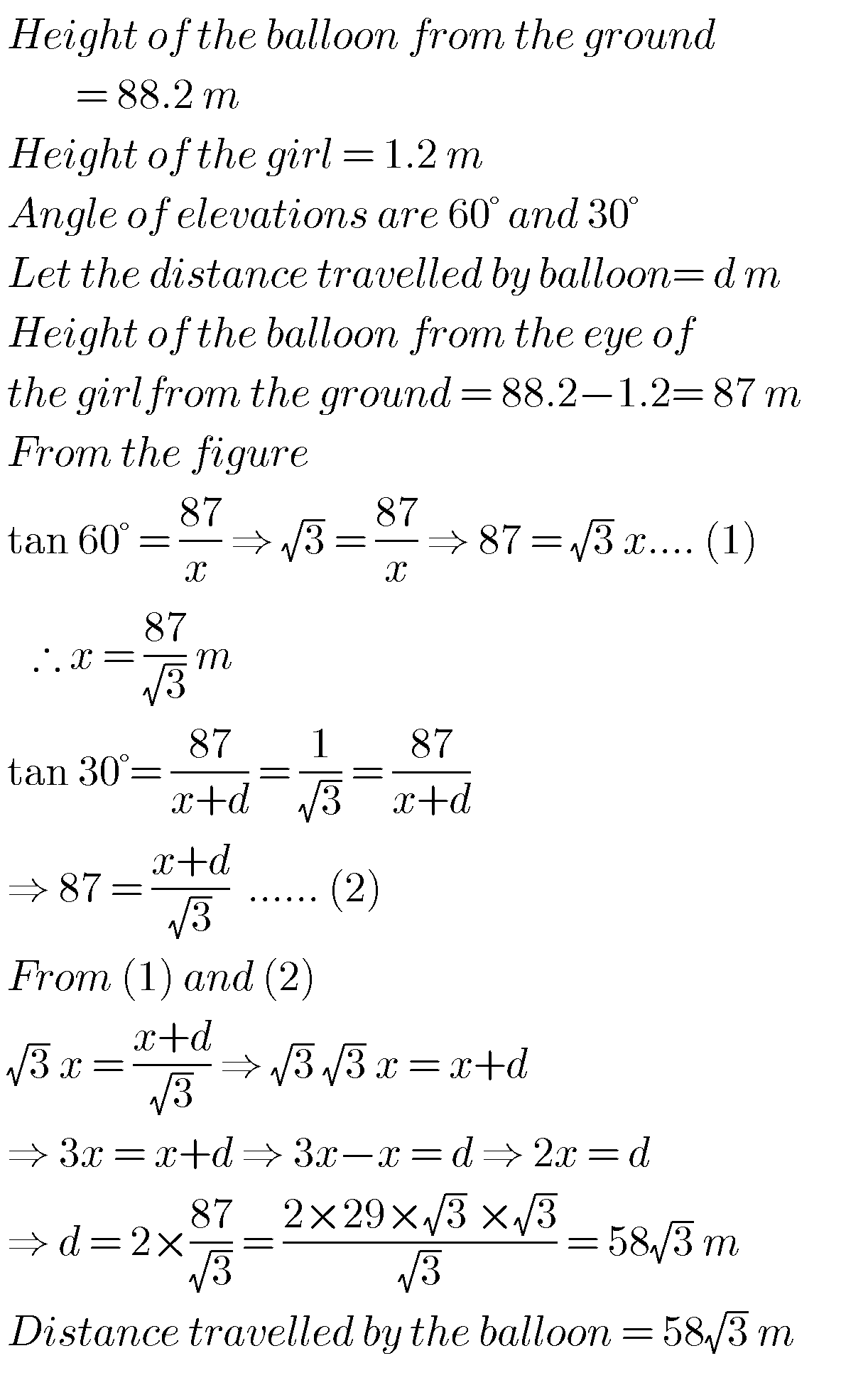## Maths solutions for Applications of Trigonometry class 10 ssc

Maths solutions for Applications of Trigonometry class 10 Exercise 12.1 and12.2 Tenth class mathematics chapter 12 exercises 12.1 and 12.2 solutions are given. These solutions are very easy to understand. First study the chapter 12 well for many times. Observe the example probems and solutions well. Try them in your own method. Next solve the …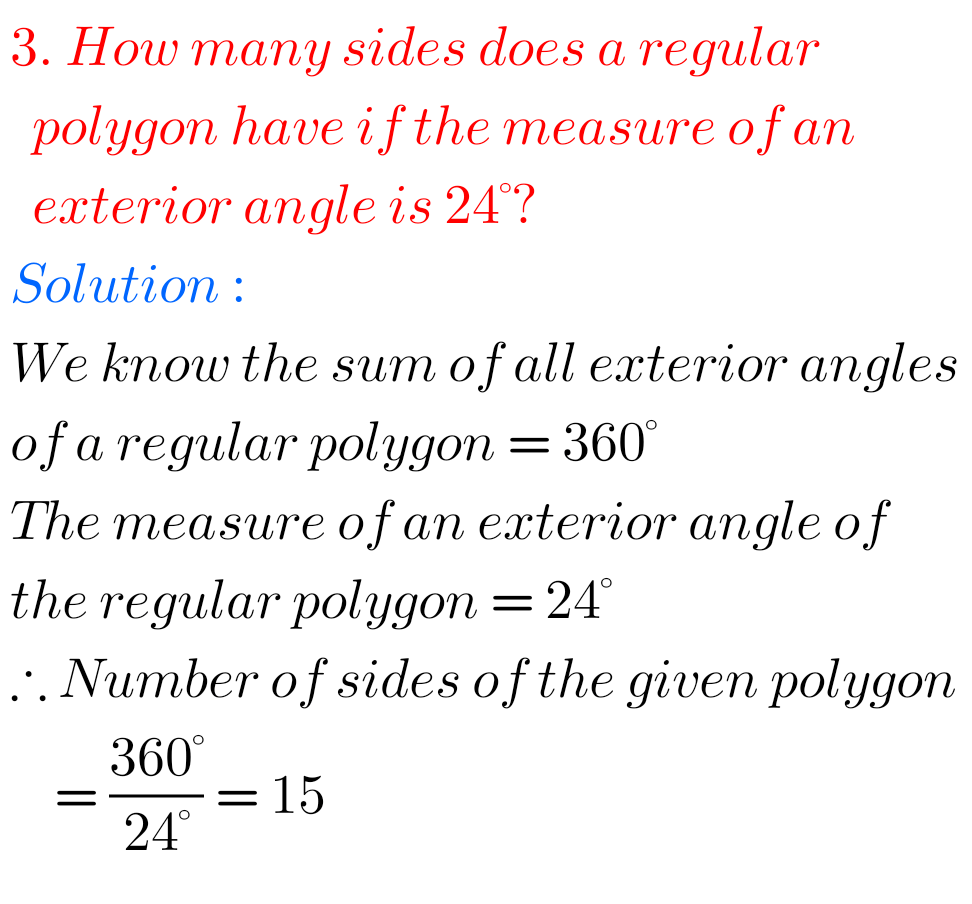## NCERT Maths solutions class 8 Exercise 3.2 Understanding Quadrilaterals

Understanding Quadrilaterals Exercise 3.2 solutions NCERT maths class 8 NCERT maths class 8 chapter 3 Understanding Quadrilaterals Exercise 3.2 solutions are given. You should study the textbook lesson Understanding Quadrilaterals very well. Observe the given below solutions and try them in your own method. NCERT maths class 8 solutions  Understanding Quadrilaterals Exercise 3.1 Exercise 3.2 …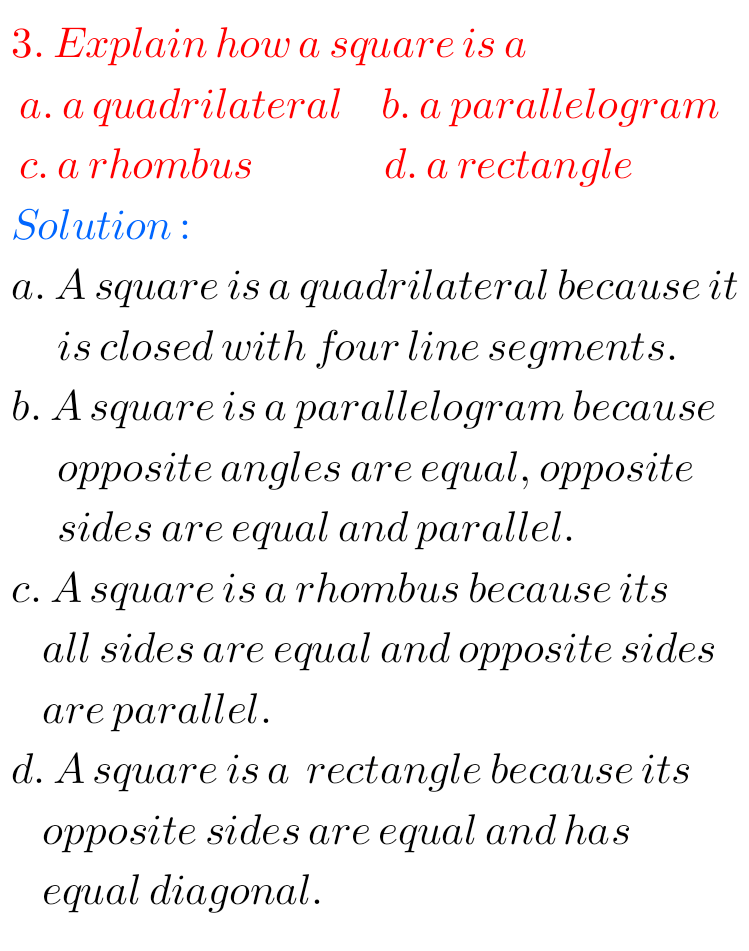## Understanding Quadrilaterals Exercise 3.4 solutions class 8 maths NCERT

Understanding Quadrilaterals Exercise 3.4 solutions NCERT maths class 8 NCERT maths class 8 chapter 3 Understanding Quadrilaterals Exercise 3.4 solutions are given. You should study the textbook lesson Understanding Quadrilaterals very well. Observe the given below solutions and try them in your own method. NCERT maths class 8 solutions Understanding Quadrilaterals Exercise 3.1 Exercise 3.2 …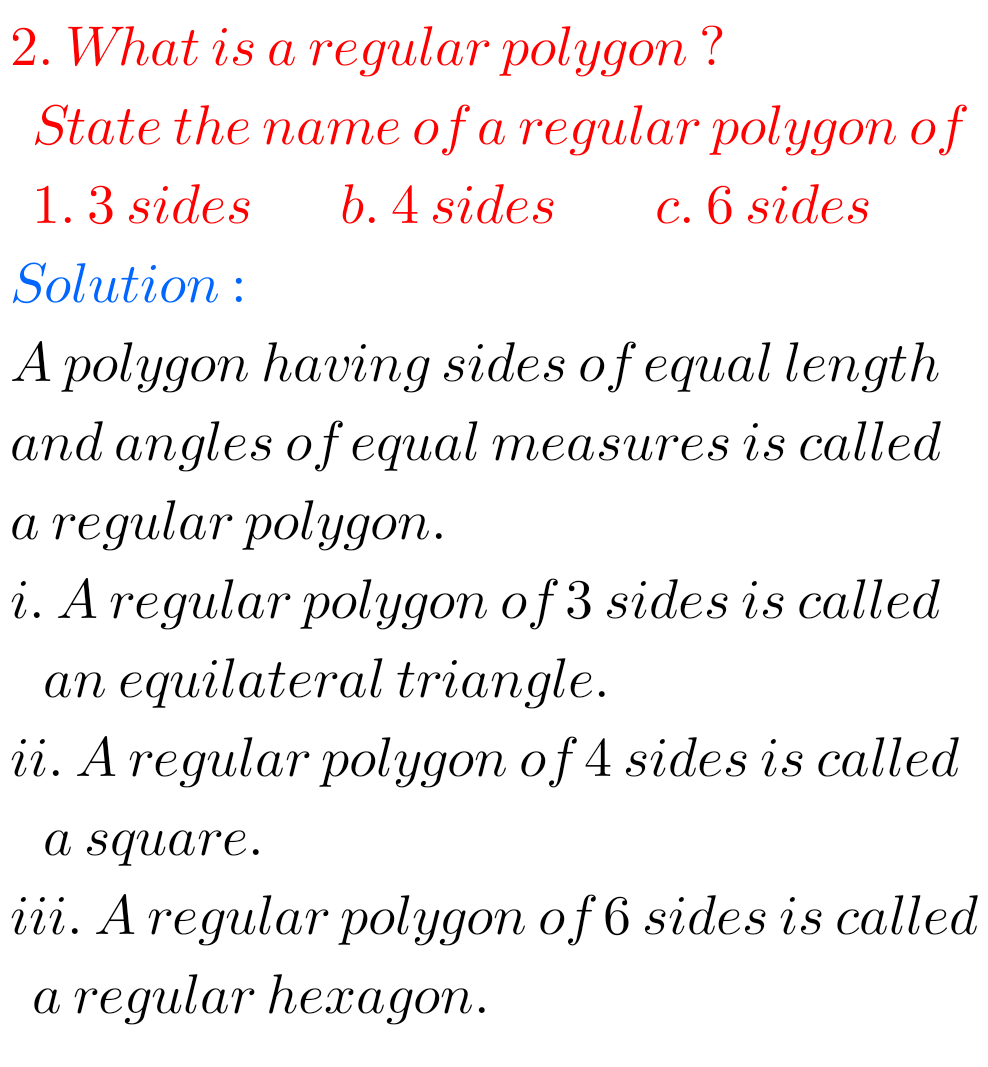## NCERT Solutions class 8 maths chapter 3 Understanding Quadrilaterals exercise 3.1

Understanding Quadrilaterals Exercise 3.1 solutions NCERT maths class 8 NCERT maths class 8 chapter 3 Understanding Quadrilaterals Exercise 3.1 solutions are given. You should study the textbook lesson Understanding Quadrilaterals very well. Observe the given below solutions and try them in your own method. NCERT maths class 8 solutions chapter 1 Understanding Quadrilaterals Exercise 3.1 …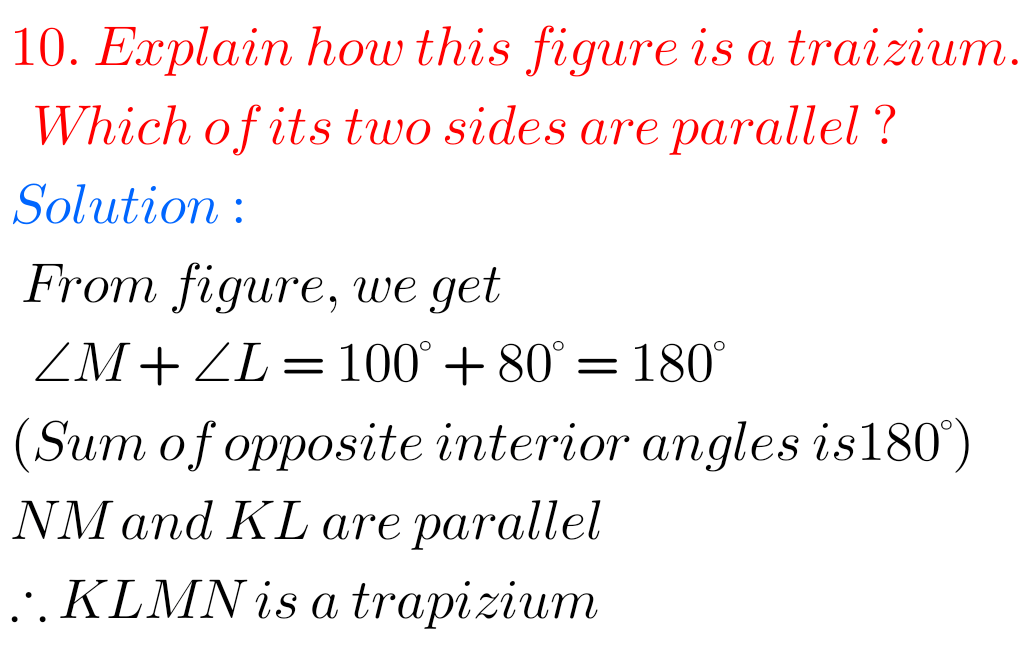## NCERT class 8 chapter 3 exercise 3.3 maths Understanding Quadrilaterals

Understanding Quadrilaterals Exercise 3.3 solutions NCERT maths class 8 NCERT maths class 8 chapter 3 Understanding Quadrilaterals Exercise 3.3 solutions are given. You should study the textbook lesson Understanding Quadrilaterals very well. Observe the given below solutions and try them in your own method. NCERT maths class 8 solutions Understanding Quadrilaterals Exercise 3.1 Exercise 3.2 …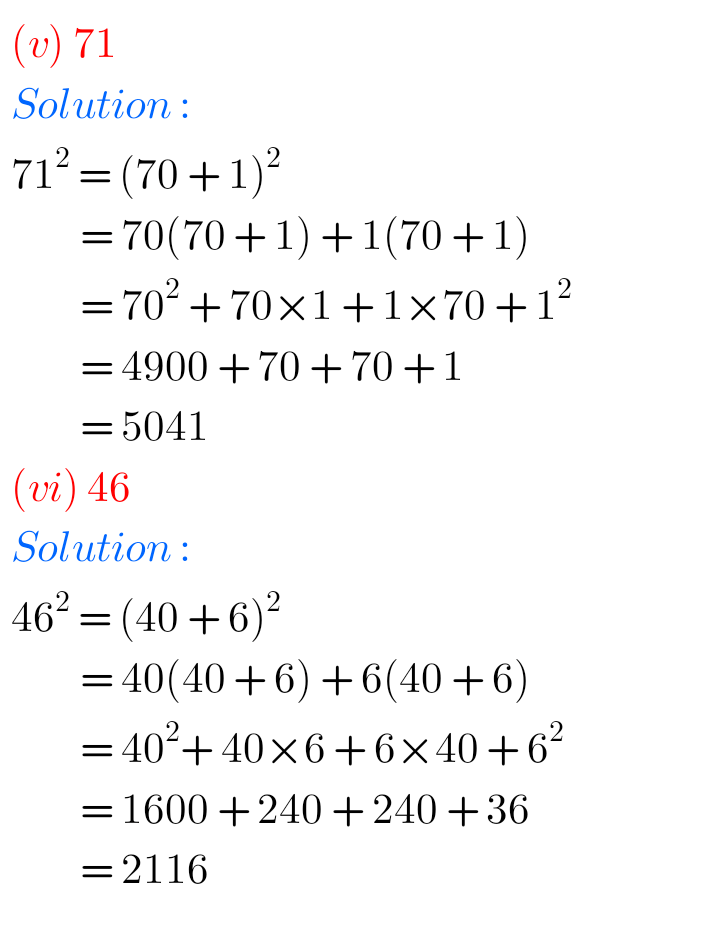## NCERT Solutions for Class 8 maths chapter 5 Squares and Square Roots exercise 5.2

Squares and Square Roots exercise 5.2 solutions Class 8 NCERT NCERT mathematics class 8 chapter 5 Squares and Square Roots exercise 5.2 solutions are given. You should study the textbook lesson Squares and Square Roots very well. You should also observe and practice all example problems are given in the textbook. Observe the given solutions …